• English：

# CS330 lecture 1&2 notes

## Informal Problem Definitions

• The multi-task learning problem: Learn all of the tasks more quickly or more proficiently than learning them independently.(更快更专业学习所有任务)
• The meta-learning problem: Given data/experience on previous tasks, learn a new task more quickly and/or more proficiently.（依照先前任务的经验，更快更好的学习新任务）

$\begin{array}{l}{\mathscr{D}=\left\{(\mathbf{x}, \mathbf{y})_{k}\right\}} \\ {\min _{\theta} \mathscr{L}(\theta, \mathscr{D})}\end{array}$

$\mathscr{L}(\theta, \mathscr{D})=-\mathbb{E}_{(x, y) \sim \mathscr{D}}\left[\log f_{\theta}(\mathbf{y} | \mathbf{x})\right]$

A task: $\mathscr{T}_{i} \triangleq\left\{p_{i}(\mathbf{x}), p_{i}(\mathbf{y} | \mathbf{x}), \mathscr{L}_{i}\right\}$

data generating distributions

Corresponding datsets:$\mathscr{D}_{i}^{tr}$ 训练集$\mathscr{D}_{i}^{t s t}$测试集 通常用$\mathscr{D}_{i}$表示训练集

Multi-task classification：$\mathscr{L}_{i}$ same across all tasks 例如：每种语言的手写识别中，损失函数的形式可能相同

Multi-label learning:$\mathscr{L}_{i}, {p}_{i}(x)$ same across all tasks 例如：CelebA 多标签识别任务中，样本和损失函数都是相同的

• mixed discrete， continuous labels across tasks（混了了离散和连续的标签的任务）

$\min _{\theta^{s h}, \theta^{1}, \ldots, \theta^{T}} \sum_{i=1}^{T} \mathscr{L}_{i}\left(\left\{\theta^{s h}, \theta^{i}\right\}, \mathscr{D}_{i}\right)$

#### Common Choices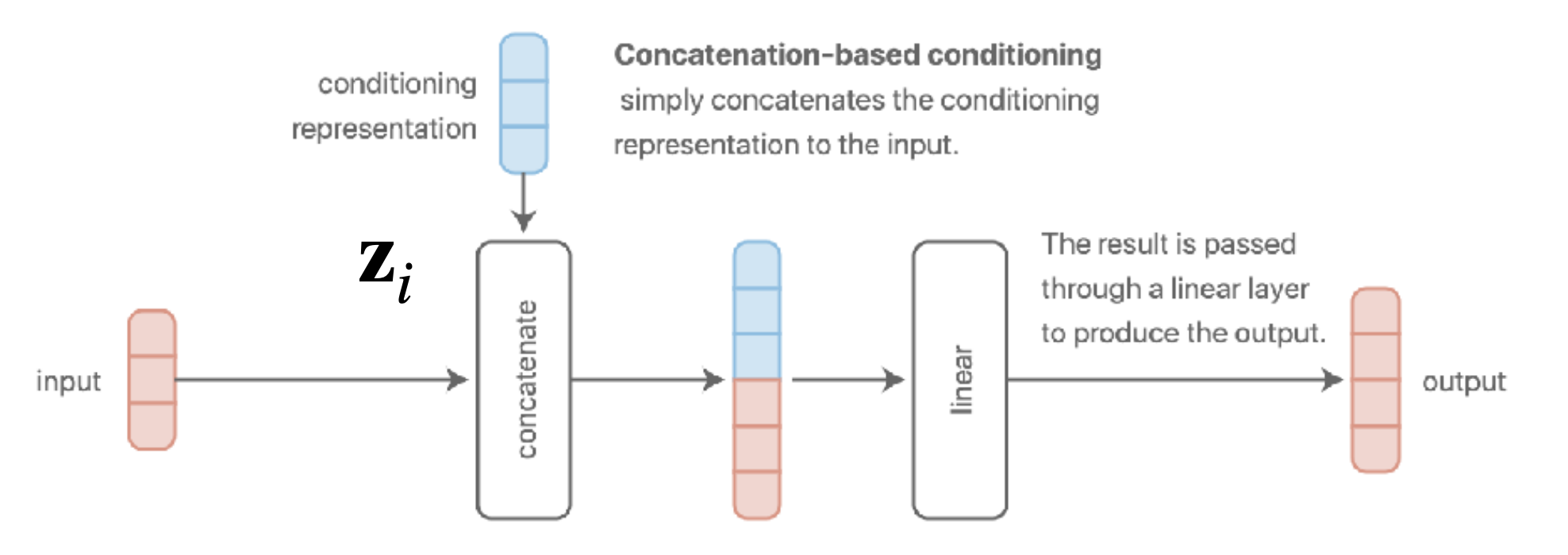cs330-1-1.png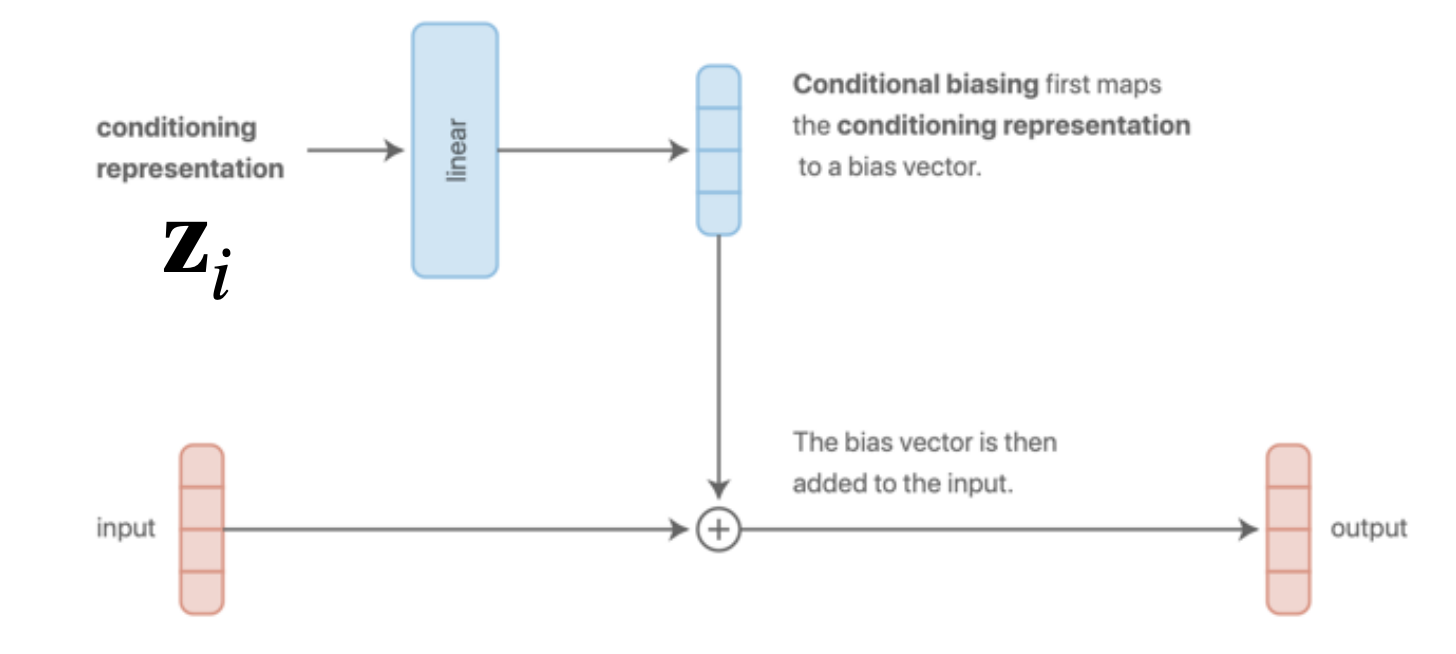cs330-1-2.png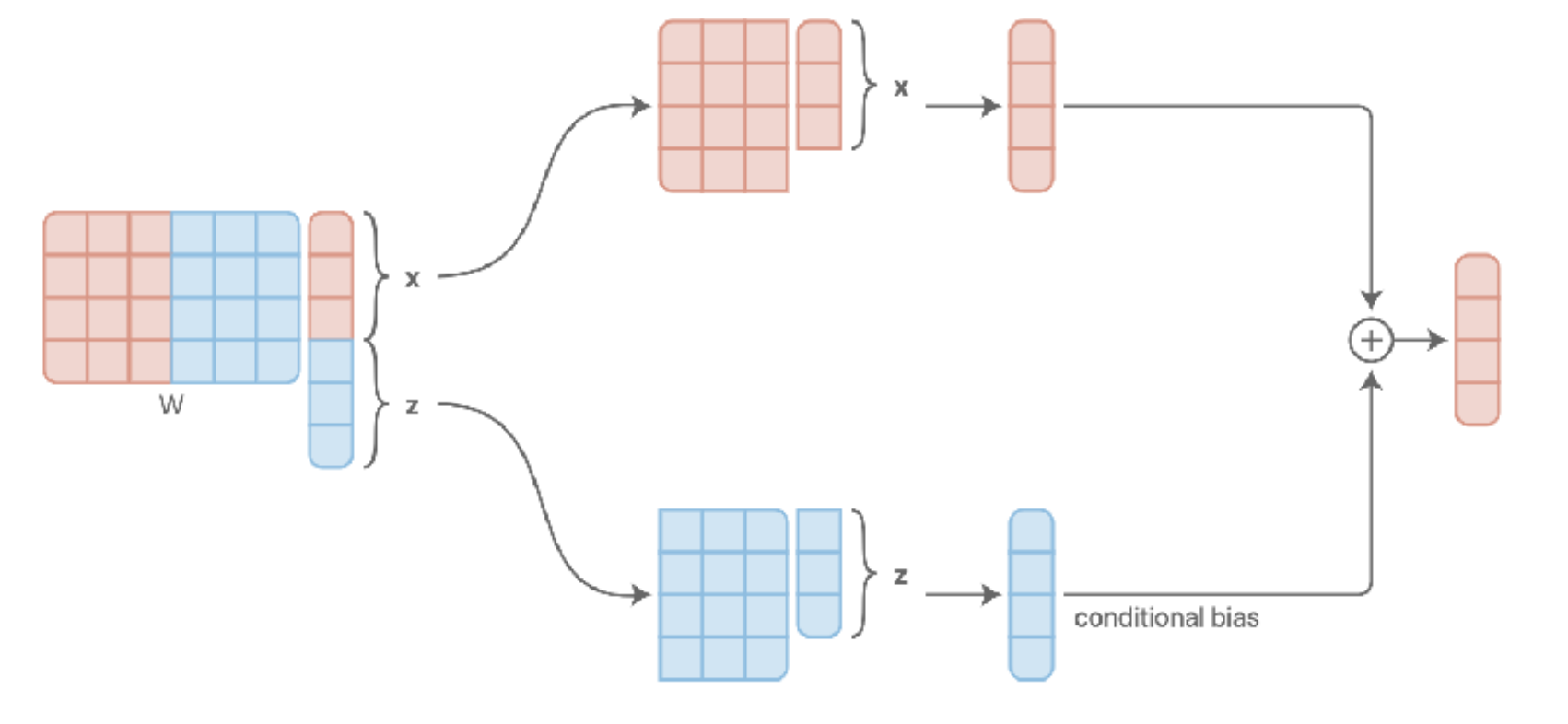cs330-1-3.png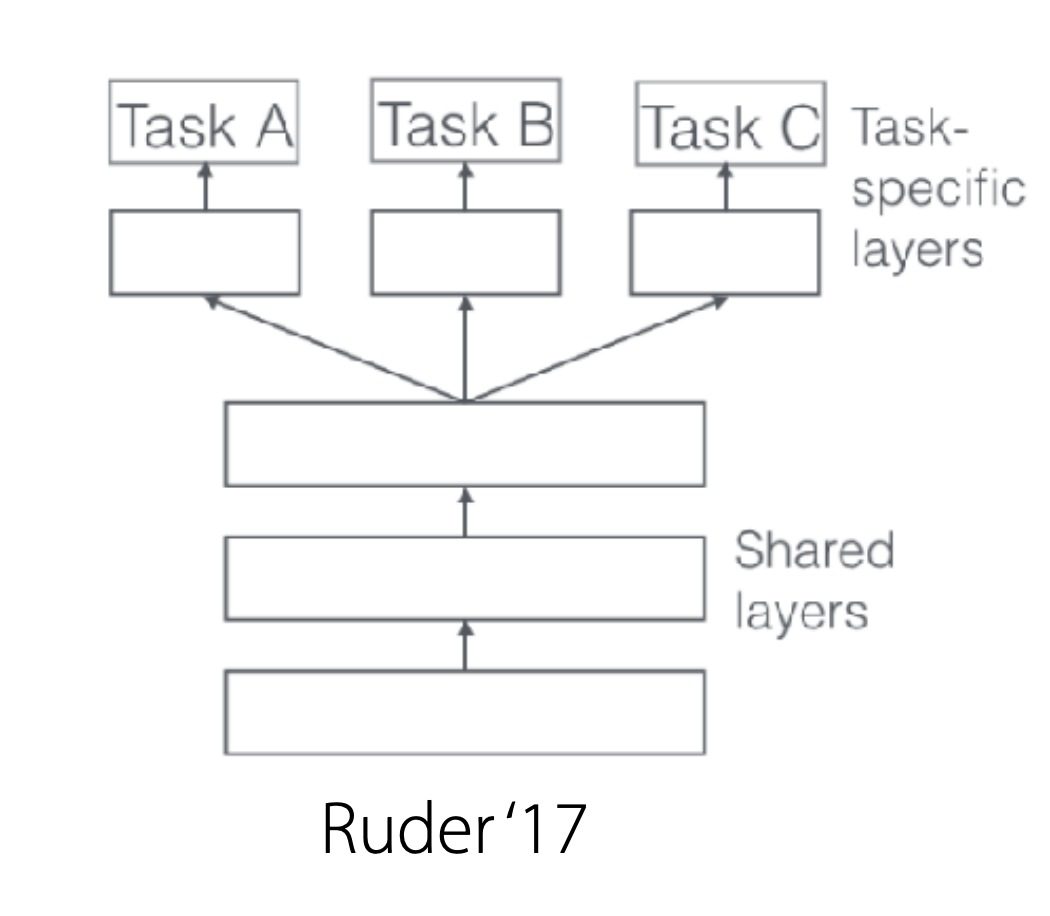cs330-1-4.png

1. Multiplicative conditioning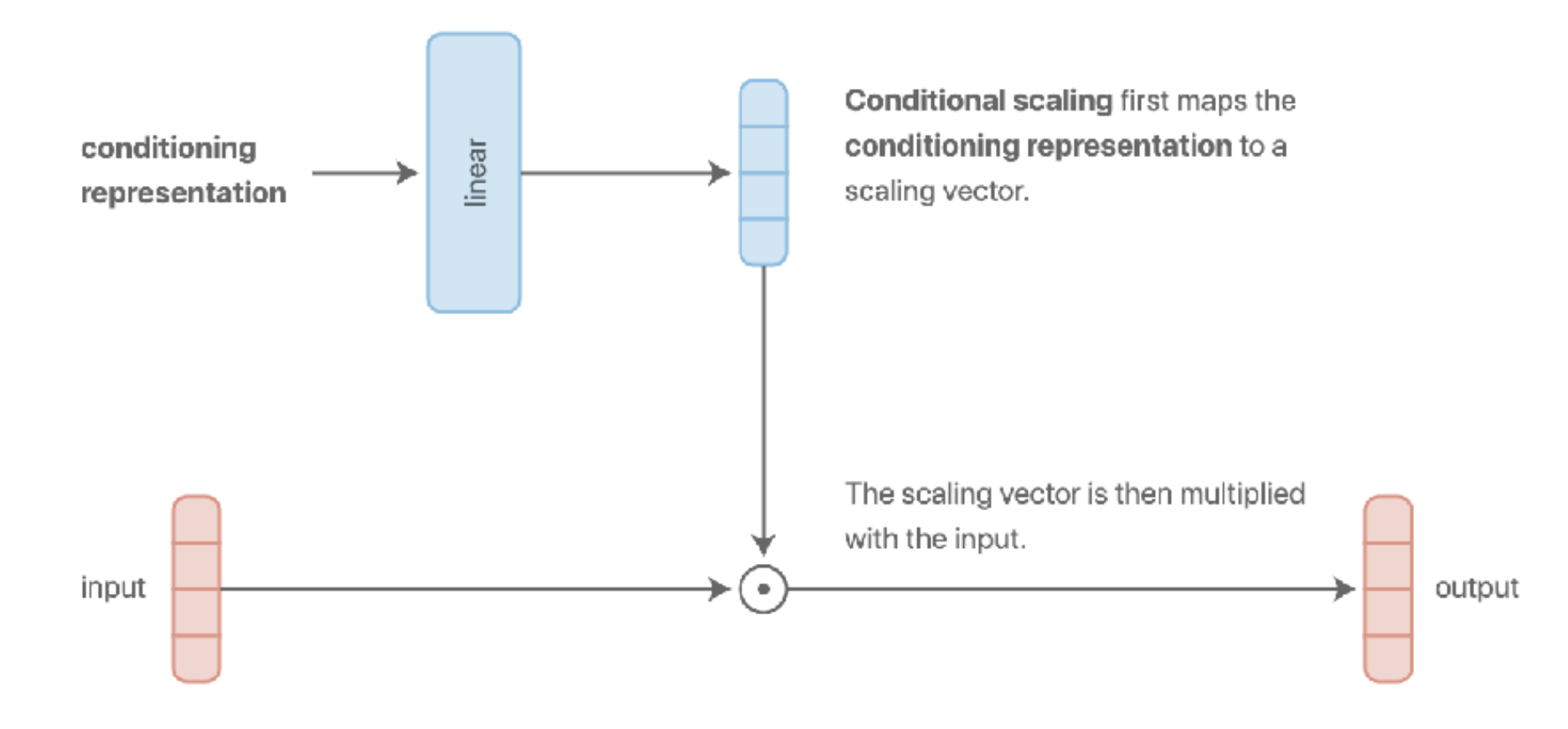cs330-1-5.png

#### Complex Choices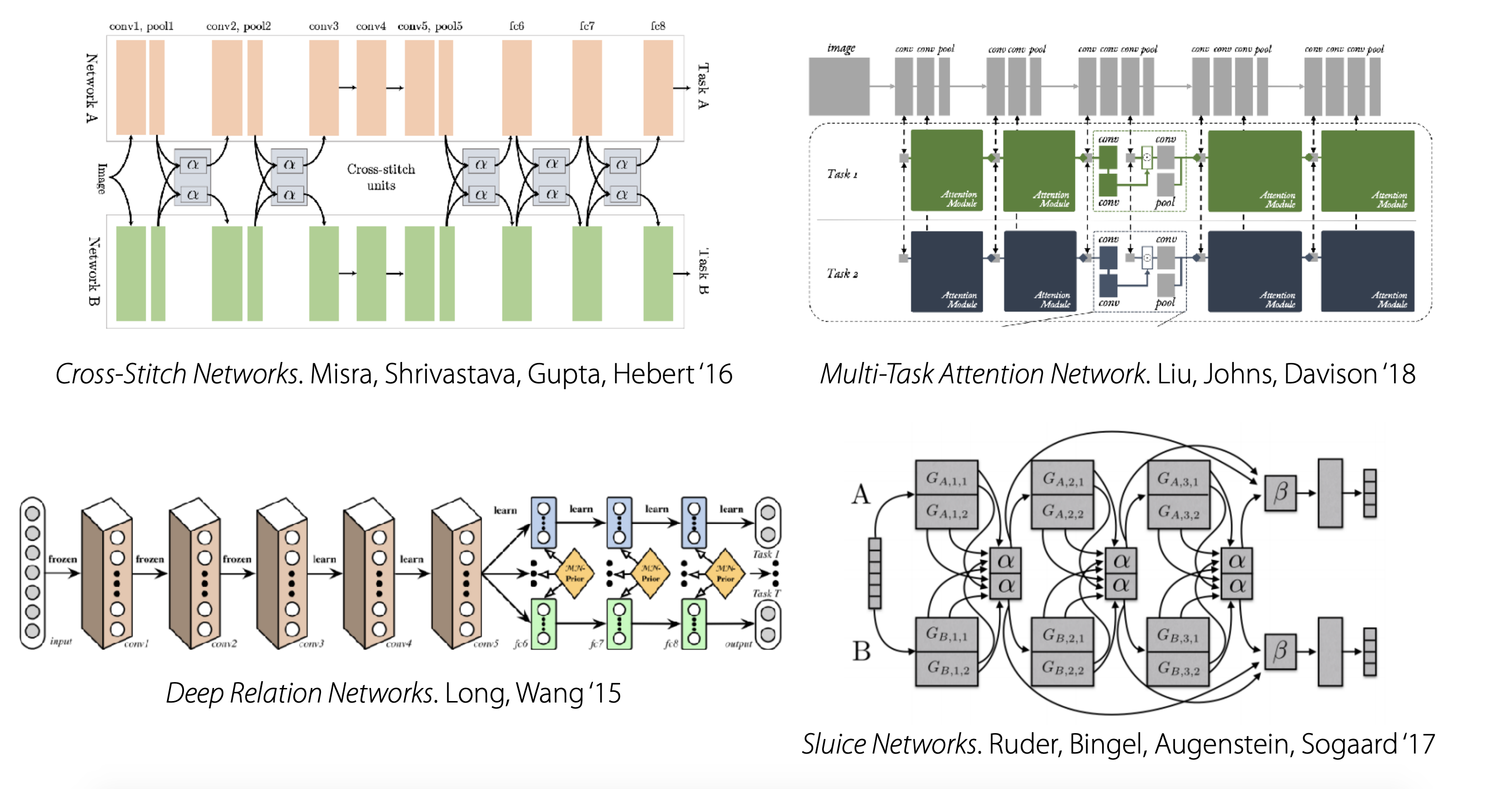cs330-1-6.png

### Optimizing the objective

Objective:$\min _{\theta} \sum_{i=1}^{T} \mathscr{L}_{i}\left(\theta, \mathscr{D}_{i}\right)$

1. 从tasks中采样一个minibatch$\mathscr{B} \sim\left\{\mathscr{T}_{i}\right\}$
2. 从每个task中采样一个minibatch的数据样本$\mathscr{D}_{i}^{b} \sim \mathscr{D}_{i}$
3. 在每个minibatch-task上计算loss：$\hat{\mathscr{L}}(\theta, \mathscr{B})=\sum_{\mathcal{T}_{k} \in \mathscr{B}} \mathscr{L}_{k}\left(\theta, \mathscr{D}_{k}^{b}\right)$
4. 反向传播计算梯度$\nabla_{\theta} \hat{\mathscr{L}}$
5. 采用你喜欢的优化器更新梯度

Note：这样可以保证无论数据量如何，任务都可以被均匀采样 Tip:对于回归任务，确保任务标签是相同的scale

### Challenge

1. Negative transfer

share less across task（软参数分享） - allows for more luid degrees of parameter sharing 好处 - yet another set of design decisions/hyperparameters 坏处

$\min _{\theta^{sh}, \theta^{1}, \ldots, \theta^{T}} \sum_{i=1}^{T} \mathscr{L}_{i}\left(\left\{\theta^{s h}, \theta^{i}\right\}, \mathscr{D}_{i}\right)+\sum_{t=1}^{T}\left\|\theta^{t}-\theta^{t'}\right\|$

1. Overfitting

To be continued